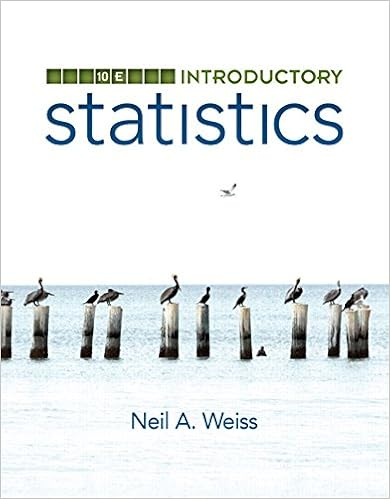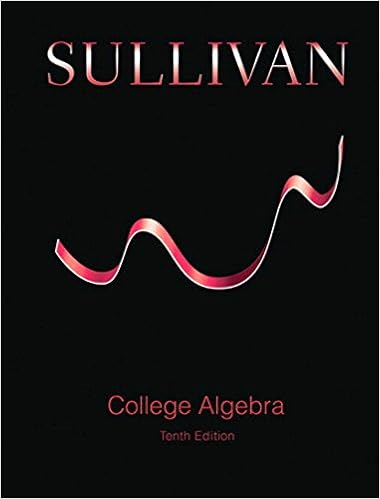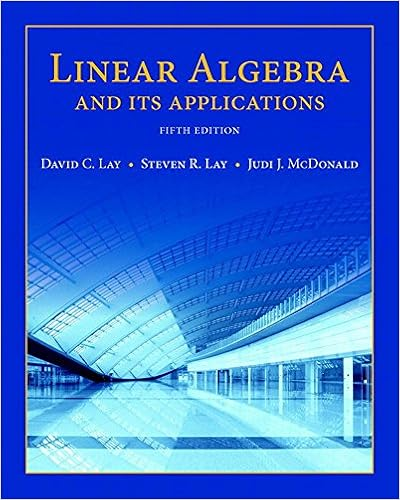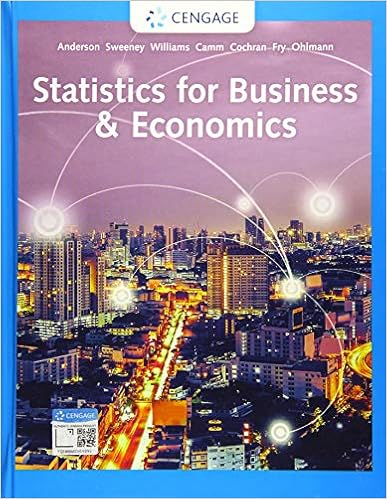# Exam C on Collge Algebra - Test Bank Exercises in CHAPTER 3...

• Test Prep
• Ramala
• 8
• 100% (1) 1 out of 1 people found this document helpful

This preview shows page 1 - 3 out of 8 pages.

The preview shows page 1 - 3 out of 8 pages.
51Exercise Set 3.11.Use the midpoint and the distance formulas, respectively, to find (a) the midpoint and (b) the dis-tance between the points (6, 5) and (–1, 4).2.Use the midpoint and the distance formulas, respectively, to find (a) the midpoint and (b) the dis-tance between the points (1/2, –3) and (1, 0).3.Use the midpoint and the distance formulas, respectively, to find (a) the midpoint and (b) the dis-tance between the points (, 5/3) and (0, 1/3).4.Given the pointsA=(–4, 3),B=(–5, 7), andC=(–1, 6), use the distance formula to find thelengthsAB,BC, andCA, and determine whether the triangleABCis (a) a right triangle, (b) anisosceles, (c) an equilateral triangle, or (d) neither.5.Give the pointsA=(1, 1),B=(–2, 4), andC=(3, 3), use the distance formula to find the lengthsAB,BC, andCA, and determine whether the triangleABCis (a) a right triangle, (b) an isosceles, (c)an equilateral triangle, or (d) neither.6.Given the pointsA=(–1, 1),B=(6, 3), andC=(1, –6), use the distance formula to find thelengthsAB,BC, andCA, and determine whether the triangleABCis (a) a right triangle, (b) anisosceles, (c) an equilateral triangle, or (d) neither.7.Given the pointsA=(1, 3),B=(8, 5), andC=(3, –4), use the distance formula to find the lengthsAB,BC, andCA, and determine whether the triangleABCis (a) a right triangle, (b) an isosceles, (c)an equilateral triangle, or (d) neither.8.Given the pointsA=(–1, 1),B=(3, 1), andC=(1, 1+), use the distance formula to findthe lengthsAB,BC, andCA, and determine whether the triangleABCis (a) a right triangle, (b) anisosceles, (c) an equilateral triangle, or (d) neither.9.Using the formulafind the area of the right triangle whose vertices are(–3, –1), (4, 1), and (–1, –8).Area12(base) (height),233Test Bank Exercises inCHAPTER3
##### We have textbook solutions for you!The document you are viewing contains questions related to this textbook.
Chapter 1 / Exercise 43
Mathematical Applications for the Management, Life, and Social Sciences
HarshbargerExpert VerifiedBrowse all Textbook Solutions
10. TheHeron’s formulasays that ifa,b, andcare the side lengths of a triangle, and 2s=a+b+c, thenthe area of the triangle isFind the area of each triangle in problems 4to 8 above.11. The area of a triangle whose vertices are the points (x1,y1), (x2,y2), and (x3,y3) is given byUse this formula to find the area of each triangle in problems 4 to 8 above.12. Three vertices of a square are (4, –1), (0, –3), and (2, 3). Find the fourth vertex.13. An end point and the midpoint of a line segment are respectively (3, –5) and (–1, 2). Find the otherendpoint.14. Without graphing, determine if the points (–1, 0), (7, 4), and (11, 6) lie on the same straight line.15. Sketch the parallelogram with vertices (–5, 1), (6, 5), (9, 10), and (–2, 6). Find the midpoints of thetwo diagonals of this parallelogram. Can you draw any conclusion from this?

Course Hero member to access this document

Course Hero member to access this document

End of preview. Want to read all 8 pages?

Course Hero member to access this document

Term
Fall
Professor
Dr.YunMyungOh
Tags
triangle, Exercise Set
##### We have textbook solutions for you!
The document you are viewing contains questions related to this textbook.The document you are viewing contains questions related to this textbook.
Chapter 1 / Exercise 43
Mathematical Applications for the Management, Life, and Social Sciences
HarshbargerExpert Verified
•••